Communications
Volume 3, Issue 1, January 2015, Pages: 1-10

Simulation of Digital Optical Receiver of Intensity Modulation and Direct Detection

Manuel Vítor Martingo Coelho

Department of Electrical and Computer Engineering, Higher Technical Institute, University of Lisbon, Lisbon, Portugal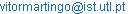Manuel Vítor Martingo Coelho. Simulation of Digital Optical Receiver of Intensity Modulation and Direct Detection. Communications. Vol. 3, No. 1, 2015, pp. 1-10. doi: 10.11648/j.com.20150301.11

Abstract: This article presents the implementation of an interactive software that integrates various functional blocks of an optical receiver of intensity modulation and direct detection (IM-DD), with OOK (on-off keying) digital modulation and NRZ (non-return-to-zero) pulse format. The software allows for the isolated simulation of each block, as well as the complete simulation of the whole system. We underline the following results presented by the simulator: theeye diagram, the probability density functions of the samples, Bode diagram, bandwidth, transimpedance gain, signal-to-noise ratio, power of the different noise sources and the bit error probability of the simulated system.

Keywords: Interactive Simulator, Optical Receiver, Optical Amplifier, Photodetector, Electrical Preamplifier, IM-DD

1. Introduction

The first optical fibre commercial transmission systems were deployed at the end of the seventies  and have gone through an exponential evolution up to the present. The reasons behind the enormous success of optic fibre transmission have to do with the following -: large bandwidth, immunity to electromagnetic interference, reduced attenuation, low cost, reduced dimensions and greater reliability.

The optical receivers can be based on direct detection orcoherent detection.

In direct detection, the photodetector of the optical receiver generates a current that is proportional to the optical power that falls on it, in which case the information, which can be either analogue or digital, is encoded into the signal intensity. When it comes to digital transmission, the modulation that is used more frequently is OOK, where, ideally, the "0" bit corresponds to the absence of light and the "1" bit corresponds to the presence of light. In this case the pulses, in the NRZ form (non-return-to-zero – thepulse occupies the whole period of the bit) orRZ form (return-to-zero – the pulse only occupies a fraction of the bit period)must be, imperatively, unipolar. The NRZ pulses are used more often because they need a lesser electrical bandwidth from the receiver. However, in the case of non-linear propagation of solitons in the optic fibre, the pulses cannot have the NRZ form due to the width characteristics of the soliton type pulse. In this case RZ encoding is used where the light pulses do not occupy more than 20% to 25% of the bit period.

In coherent detection, information arrives at the optic receiver modulated on a carrier wave by "ASK" (Amplitude-Shift Keying), "FSK" (Frequency-Shift Keying) or "PSK" (Phase-Shift Keying). The coherent detection can also be heterodyne or homodyne. In both cases the receiver needs a local optical oscillator (laser diode), where the output is adequately "mixed" with the received optical signal so as to obtain the information contained in the optical carrier.

Although good results have been obtained through coherent detection, direct detection is used more widely due to its simplicity. The option for direct detection was further promoted by the emergence of optical amplifiers, which bridged some of the weaknesses of this type of detection .

Although there are presently simulators for optical communications systems, as are the case of the Optiwave, VPIphotonics simulators and also the OptSim simulator from Rsoft, the development of new, easily accessible tools which are focused on certain more specific aspects of the study are, always, an added value to education, research and development in this area.

2. Simulator

2.1. Developing the Simulator

The simulator was developed in four distinct phases: Analysis, Design, Implementation and Testing. The simulator was developed in Matlab language, also known as M-code, since it is a simple and very popular language in the education andresearch areas.

The simulator can be run on computers with different operating systems (Windows, Linux, Solaris and Mac). To this effect, all you have to do is to launch the installation file "MCR" (Matlab Compiler Runtime). After the installation the computer does not need any version of Matlab to run the simulator.

2.2. Operating the Simulator

The program starts with a Welcome window (Fig. 2.1) representing the simulator’s main menu.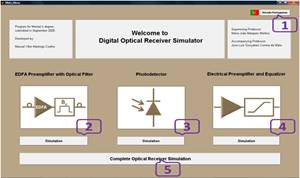There are five buttons available on this menu, which are numbered 1 to 5 in Fig. 2.1,for easy reference:

Button 1 → Change of version (in this case, to the Portuguese version);

Button 2 → Isolated simulation of the EDFA preamplifier with optic filter;

Button 3 → Isolated simulation of the photodetector;

Button 4 → Isolated simulation of the electrical preamplifier and the equalizer;

Button 4 → Complete simulation of the optical receiver.

When the user presses any of the buttons (with the exception of the change of version button) a new window opens where the requested simulation can be run.

The windows of the different simulations will be displayed during the course of this article.

3. Optical Preamplification

3.1. Optical Amplifier

The two main types of optical amplifiers are: semiconductor optical amplifiers (SOA) and doped-optical fibre amplifiers (DFA) .

The semiconductor optical amplifiers operate identically to the semiconductor lasers and present worse amplification characteristics than the doped-optical fibre amplifiers.

The doped-optical fibre amplifiers are obtained by doping an optic fibre with chemical elements belonging to the "rare earth" group. The optical amplifier most used in recent years is the EDFA (Erbium Doped Fibre Amplifier) due to the simplicity of its manufacture, its easy coupling to the fibre and also due to the fact that its amplification spectrum coincides with the minimum of attenuation of the optic fibres, which corresponds to "1550 nm" . The EDFA uses erbium in its ionic form (Er+3) to dope the fibre.

3.2. Optical Amplifier – EDFA

The EDFA amplifier consists of a section of silica fibre with a nominal length ranging from 10 m to 30 m, doped with erbium ions (e.g. 1000 ppm). The doped fibre is pumped through a laser pump with a wavelength of 980 nm or 1 480 nm.

3.2.1. EDFA Gain

According to ITU (International Telecommunications Union), 35 nm (from 1 530 nm to 1 565 nm) is considered to be a useful band of amplification of the EDFA. On the other hand, with the increase in power of the input signal, the EDFA gain diminishes. This effect is called gain saturation, and it occurs when the population inversion is significantly reduced due to the high number of photons of the input signal. So therefore, the EDFA gain may be expressed through the conservation of energy principle: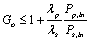(3.1)

where "G0" represents the optical gain of the amplifier,"λp" is the wavelength of the laser pump, "λs" is the wavelength of the signal, "Pp,in" the maximum power of the laser pump and "Ps,in" is the power of the EDFA input signal.

3.2.2. EDFA Noise

During the EDFA amplification process, the amplified spontaneous emission(ASE) takes place and some photons are transmitted when the carriers pass spontaneously to their fundamental level. These photons appear as noise at the amplifier output.

Since there are two polarisation modes in the optic fibre and admitting that the noise power distributes itself equally in the two modes, the total noise power is given by :(3.2)

where "PEAA" is the noise power for each polarisation mode, "SASE" represents the spectral density of the noise power, "B0" is the optical bandwidth, "nsp" is the spontaneous emission factor, "G0" is the amplifier optical gain, "h" is the Planck constant and "v"the frequency.

The noise introduced by the optical amplifier is usually specified through the "F0" noise factor parameter :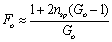(3.3)

When the gain of the optical amplifier is high, the expression (3.3) can still be simplified into: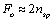(3.4)

Replacing the expression (3.4) in (3.2) we obtain: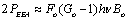(3.5)

The optical signal-to-noise ratioat the output of the optical amplifier can be defined by: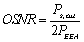(3.6)

where "OSNR" represents the optical signal-to-noise ratio. Bearing in mind that "Ps,out=G0Ps,in" and replacing the expression (3.5) in (3.6), we finally obtain:(3.7)

3.3. Optical Filter

As a form of reducing the power of the optical noise inserted by the optical amplifier, in the optical preamplifier, an optical filteris normally used an optical filter between the amplifier and the photodetector.The optical filter reduces the optical bandwidth and, consequently, reduces the power of the optical noise.

The power transfer function of the optical filter is designated as transmittance. In the case of the simple cavity Fabry-Perot filter, the transmittance "TFP(f)", is given by :(3.8)

where"R" represents the mirror reflectivity and "FSR" thefree spectral range which corresponds to the optical spectrum that exists between a given wavelength and its multiple. The transfer function of the optical filter is a periodical function in frequency, with a period equal to the free spectral range.

3.4. EDFA Preamplifier and Optical Filter Simulation

The individual simulation of the optical preamplifier (Fig. 3.1), represented by an EDFA and an optical filter, allows the signal at the input, the connector used, the intrinsic parameters of the EDFA and the optical filter to be scaled.

The variable parameters of the incident optical signal and connector are formed by the average optical power that reaches the connector, the path penalty, the wavelength, the bit rate, theextinction ratioand the optical connector losses.The value of the extinction ratio "r" corresponds to the ratio between the optical power of the logical level "0" and the optical power of the logical level "1":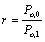(3.9)

Since it is considered that in OOK digital modulation "Po,0<Po,1", then the extinction ratiovaries between "0<r<1".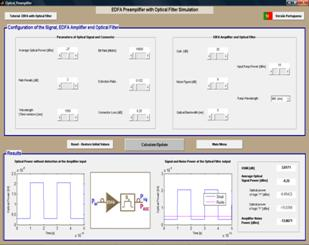Figure 3.1. Simulation of the EDFA preamplifier with optical filter.

It must be stressed that, in this simulation, the wavelength is limited to the third window or C band (between "1530nm" and "1565nm"), due to the limitations of the EDFA amplifier.

With relation to the EDFA and the optical filter, the individual simulator allows the scaling of the following parameters: gain, noise figure,optical filter bandwidth, power of the laser pump and the wavelength used in pumping.

In the results that are presented, the simulator uses the expressions above to process the variable parameters. The simulator presents graphically the results of the NRZ optical signal at the optical amplifier input (according to the defined parameters), as well as the signal power and noise power at the optical filter output. Values resulting from the optical signal-to-noise ratio, average signal power, logical level "1" power and the logical level "0" powerand amplified spontaneous emission factorpower brought in by the EDFA are shown.

4. Photodetector

The photodetector is the element of the optical receiver that is responsible for converting the signal from the optical domain to the electrical domain, by means of the photo-electrical effect. Notwithstanding the diversity of photodetectors that exist, the PIN (Positive-intrinsic-Negative) and APD (Avalanche Photodiode) photodiodes are used, almost exclusively, in optical communications. These present improved characteristics, due to their reduced size, high sensitivity, fast response in time and low cost.

4.1. PIN Photodiode

The PIN photodiode consists in a junction of "p-n" materials, separated by an intrinsic material.

Ideally, the PIN photodiode originates a pair of electron-hole for each incident photon. However, in truth, not all incident photons in the semiconductor material are absorbed and, as a consequence, cannot generate electron-hole pairs. The opto-electrical conversionefficiency is called quantum efficiency, "η", and may be obtained by the following expression :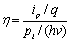(4.1)

where "ip" is the electric current at the PIN photodiode output, "q" is the electric charge of an electron, "pi" is the optical power of the incident signal in the photodetector and "hv" is the energy of each photon. The quantum efficiencydepends on the wavelength of the incident optic signal, as well as the type of material used in manufacturing the photodetector.

Another usual and very useful value that characterises the photodiode is its responsivity, "R0", which translates the relationship between the generated current and the incident power in the PIN photodiode :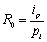(4.2)

By replacing the expression (4.1) in (4.2), we obtain the relationship betweenquantum efficiencyand responsivity: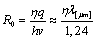(4.3)

By analysing the expression (4.3) we can observe that the responsivityincreases with the wavelength, since there are more photons for the same incident optical power. However, from the critical wavelength "λc" on, the quantum efficiencyand the responsivitylowers to zero, since the material stops absorbing the incident optical power.

4.2. Avalanche Photodiode (APD)

The APD is a photodiode with the capacitance to internally amplify the current generated during the photodetection process. The APD differs from the PIN photodiode due to the high polarisation voltages and the addition of one more type "p" layer in its structure. The APD operation is based on the ionisation by impact. The signal current at the APD output, "iAPD", is amplified by an "M" factor (avalanche gain) with relation to the primary current "ip" (current which corresponds to the primary carriers):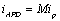(4.4)

The designation APDresponsivity, "RAPD", appears frequently in the manufacturers’ catalogues, corresponding to the ratio between the current at the APD output and the incident optical power in the same. The ratio between APDresponsivity and responsivity for the primary current "R0" is given by: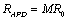(4.5)

4.3. Photo Detection Noise without Optical Preamplification

4.3.1. Quantum Noise

The generated photocurrent presents a random component "iq(t)", called quantum noise (also known as shot noise). Normally, in order to analyse the performance of an optical receiver we approach the quantum noise distribution with a Gaussian distribution with a zero mean value.

The photodiodes also generate a small current called dark current, which has its origin in the generation of electron-hole pairs through thermal effect. The contribution of this current may be included in the quantum noiseof the photodiode. Therefore the current variance of the quantum noise, "σq2", which corresponds to the mean-square value of the respective current, "˂iq2˃", at the photodetector output is given by :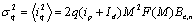(4.6)

where "q" is the electron charge, "ip" is the primary current at the photodetector output, "Id" is the dark current of the photodiode, "Be,n" is the equivalent noise bandwidth of the electrical part of the optical receiver (obtained from the transfer function of the circuit) and, finally, "F(M)" is the excess noise factor of the APD, which can be obtained from , , :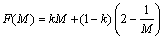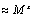(4.7)

where "k" is an adimensional parameter for the different types of semi-conductor materials, which represents the ratio between ionisationby impactcoefficients of the electrons and the holes, or vice-versa, so that "0<k<1"; on the other hand, "x" represents a parameter that is characteristic of the type of the photodiode material which is obtained from experimental results.

The expression (4.6) for calculating the APDquantum noise can be customised for the PIN photodiode, as long as "M=1" is considered.

Bearing in mind the expression (4.2) and replacing it in the expression (4.6), we obtain the current variance of quantum noise in function of the incident optical power on the "pi" photodetector: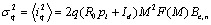(4.8)

4.3.2. Circuit Noise

The circuit noise comes from the resistive and active elements that are present in the optical receiver. For the isolated simulation of the photodetector we consider a circuit composed only by the photodiode and by a load resistor, "Rb", as we can see in Figure 4.1.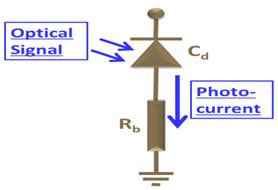Figure 4.1. Polarisation circuit of the photodiode.

In this case, the circuit noise originates only from the thermal noise of the resistor, "Rb" (also referred, as Jonhson or Nyquist noise). Therefore, the current variance of the circuit noise of Fig. 4.1, "σc2", which corresponds to the mean-square value of the circuit noise current, "˂ic2˃", is given by :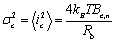(4.9)

where "kB" is the Boltzmann constant and "T" the absolute temperature (Kelvin).

4.4. Photo Detection Noise with Optical Preamplification

The photodetection of a signal with optical preamplification is carried out through a photodiode without internal gain of the PIN type. When the optical signal is amplified before it is detected, it reaches the photodetector, already corrupted by the amplified spontaneous emission noise (ASE) that was introduced by the optical amplifier. During photodetection the ASE optical noisepower is converted into the electrical domain in parallel with the signal optical power. As a consequence, new forms of noise appear: signal-ASE beat andASE-ASE beat. Although the circuit noise remains constant, the variance of the quantum noise current is now calculates from :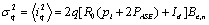(4.10)

where "2PASE" is the total ASE noise given by the expression (3.5), which also contributes to the quantum noise.

On the other hand, the variances of thesignal-ASE beat noise 2s-ASE", andASE-ASE beat noise, 2ASE-ASE", currents, which correspond to the mean-square values of the corresponding currents, "˂i2s-ASE˃" and "˂i2ASE-ASE˃", are given by :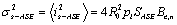(4.11)(4.12)

where "R0" is the responsivity, "SASE" is the spectral density of ASE noise power for each polarisation mode, "B0" is the optical bandwidth at the photodetector input and "Bbe,n" is the equivalent noise bandwidth of the electrical part of the optical receiver.

It is assumed that the different forms of noises have a Gaussian distribution with zero mean value. Since the different types of noise are not correlated, the variance of the total noise current, "σn2", which corresponds to the mean-square value of the total noise current, is obtained from the sum of the different noise variances referred before: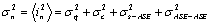(4.13)

4.5. Photodetector Simulation

The individual simulation of the photodetector (Fig. 4.2) makes it possible to scale the incident optical signalin the photodetector as well as the intrinsic parameters and the load resistor.Figure 4.2. Photodetector Simulation.

The variable parameters of the incident optical signal are made up by the average optical power, the wavelength,Bit Rate , extinction ratio and, in case the signal has been submitted to optical preamplification, the average noise power introduced by the amplifierand optical bandwidth of the amplifier or the optical filter.

With relation to the photodetector, the individual simulator allows the type of photodiode, the scaling of its responsivity, its avalanche gain (in the case of APD), the corresponding dark current, the type of material, theelectrical capacitanceof the photodiodeand the load resistor to be chosen. The simulator presents immediately bandwidth at -3dB and the equivalent noise bandwidth.

In the results that are presented, the simulator lets us view graphically the NRZ optical signal at the photodetectorinput (according to the defined parameters), the structure of the polarisation circuit of the photodiode, as well as the graphic of the photocurrent generated. The values resulting from the photocurrent are also presented with a mention of the existence or absence of inter-symbolic interference (ISI), as well as the signal-to-noise ratio, bearing in mind the power penalty due to the extinction ratio, the value of the power of the different types of noise (circuit, quantum, signal-ASE beat andASE-ASE beat) the optical powers of the "0" and "1" logical levels and the corresponding generated photocurrents.

5. Electrical Preamplifier

Usually the signal at the photodetector output is very weak and it needs a low noise amplification in order to be adequately processed. The electrical preamplifier changes the small photocurrent generated by the photodiode into voltage. Since noise increases with bandwidth, there must be a compromise between the two in order to optimize the performance of the receiver. The three most used configurations of the preamplifier are: voltage amplifier (also known as low impedance amplifier), high impedance amplifier and transimpedance amplifier.

5.1. Voltage Preamplifier

The voltage preamplifier, or low impedance preamplifier, has the structure represented in Fig. 5.1.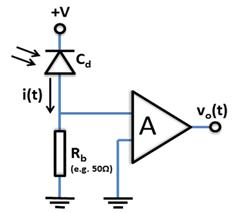Figure 5.1. Structure of the voltage(or low impedance)preamplifier.

In this type of configuration the current generated in the photodetector originates a voltage at the terminals of the resistor "Rb" (with typical values around 50Ω). This voltage is amplified by the operational amplifier with an "A" open-loop gain.

In practice, the photodetector has a parasite capacitance associated with its junction ("Cd") and the amplifier has aninputimpedance given by the parallel combination of "Ra" and "Ca". Therefore, the transfer function of the circuit in Fig. 5.1 is given by the following expression:(5.1)

where "RT" is the total resistance obtained from "RT =Rb//Ra=(RbRa)/(Rb+Ra)"; on the other hand, "CT" represents the total capacitance of the circuit which is obtained from "CT= Cd//Ca= Cd+Ca".

The value of the resistance "Rb" is fundamental for the value of the circuit noise coming from this type of preamplifier configuration.

When the circuit noisecurrent ("ic") passes through the electrical preamplifier it is translated into a noise voltage ("vc"), through the transimpedance gain of the electricalpreamplifier (obtained from the low frequency transfer function). Therefore, the variance of the circuit noisevoltage,c2", which corresponds to the mean-square value of the circuit noise voltage "˂vc2˃", at the voltage preamplifier output, is obtained from: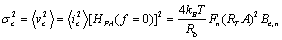(5.2)

where "Fn" is the noise factor of the amplifier (since the electric components that are present in the operational amplifier also contribute to the circuit noise) and "RTA" is the transimpedance gain of the voltage preamplifier, obtained from the expression (5.1) for "f=0".

5.2. High Impedance Preamplifier

The high impedance preamplifier has a structure that is very similar to the configuration of the voltage amplifier mentioned earlier. However, this configuration includes, also, an equalizer, as can be seen in Fig. 5.2.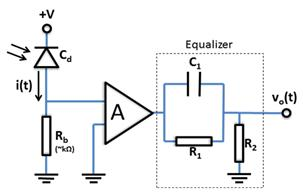Figure 5.2. Structure of the high impedance preamplifier.

The high impedance preamplifier is based on the same principle as the voltage amplifier.However, in this case, the resistor "Rb" has high values (in the kΩ range) guaranteeing a low thermal noise generated by the same. However, this type of configuration requires the use of an equalizer to compensate the reduction in bandwidth derived from the increase of the resistor "Rb". The transfer function of the circuit of Fig. 5.2 is given by the following expression:(5.3)

When scaling the equalizer, it is necessary that the equalizer zero matches the preamplifier pole, that is, "R1=RT" e "C1=CT" in order to obtain a perfect equalising.

The high impedance preamplifier with equalizer is less noisy than the voltagepreamplifier, since the resistor "Rb" has higher values. The noise introduced by the resistive elements of the equalizercan be discarded, since the signal, when it reaches the equalizer, is already amplified. Therefore, the voltage variance of the circuit noise,c2", which corresponds to the mean-square value of the circuit noise voltage "˂vc2˃", at the equalizeroutput, is obtained from:(5.4)

where"RTA[R2/(R1+R2)]" is the transimpedance gain of the high impedance preamplifier with equalizer, which is obtained from the expression (5.3) for "f=0".

5.3. Transimpedance Preamplifier

The transimpedance preamplifier has the structure presented in Fig. 5.3.Figure 5.3. Structure of the transimpedance preamplifier.

This type of configuration was developed to obtain a large bandwidth with low noise.

Taking into account the capacitance of the photodiode, "Cd", as well as the input impedance of the amplifier("Ra" and "Ca"), the transfer function for this type of configuration is given by :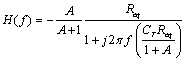(5.5)

where the equivalent resistance "Req" is obtained from: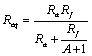.6)

Observing the previous expression we can see that if the open-loop gainof amplifier "A" is high, then "Req≈Rf".

Just as with the previous configurations, the electrical components present in the operational amplifier also contribute to the circuit noise. The noise factor of the amplifier, "Fn", is used to quantify this noise. Therefore, the variance of the voltage of the circuit noise, "˂vc2˃", at the output of the transimpedance preamplifier is obtained from:(5.7)

where "-A/(A+1)Req" is the transimpedance gain, obtained from the expression (5.5) for "f=0".

5.4. Simulation of the Electrical Preamplifier

The isolated simulation of the electrical preamplifier (Fig. 5.4) allows the optical signal at the photodetector output to be scaled, as well as the intrinsic parameters of the electrical preamplifier and equalizer.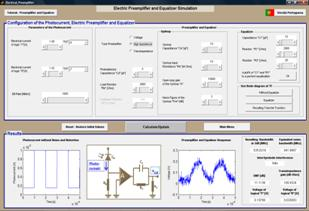Figure 5.4. Simulation interface of the electrical preamplifier.

The variable parameters of the signal at the photodetector output are the photocurrent value for "0" and "1" logical levels and the corresponding bit rate.

With relation to the electrical preamplifier parameters, the individual simulator allows for the choice of the type of amplifier (voltage, high impedance or transimpedance), the junction capacitance of the photodiode, load resistor, feedback resistance, amplifier input capacitance,amplifier open-loop gain, amplifier noise figureand the values of the components of the equalizer("R1", "R2" and "C1"). The simulator allows for the automatic adjustment of the equalizer values to a perfect equalization and makes it possible to display theBode diagram, according to the transfer functionresulting from the type of amplifier chosen and the values introduced.

In the results that are presented, the simulator shows graphically the NRZelectrical signal at the electrical preamplifierinput (according to the defined parameters), the circuit structureaccording to thechosen type of configuration, as well as the graphic of the resulting voltage at the output of the preamplifier orequalizer. The values resulting from the bandwidth at -3dB, the equivalent noise bandwidth, the mention if there is or no inter-symbolic interference (ISI), the signal-to-noise ratio (bearing in mind the penalty due to the extinction ratio), the transimpedance gain and the voltages generated for the "0" and "1" logical levels, are also presented.

The digital optical receiver, in addition to converting information from the optical domain into the electrical domain, also has the function of processing adequately the electric signal in order to recover the transmitted digital information with the minimum possible error.

Fig. 6.1 prepresents a simplified block structure of a digital direct detection optical receiver with optical preamplification.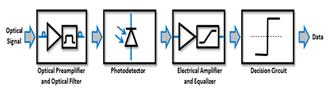Figure 6.1. Simplified block structure of a digital optical receiver with optical preamplification.

The performance of the optical receiver in digital transmission systems as is the case, is evaluated by the bit error probability, "Pe", which corresponds to the probability of incorrect decision or identification of a bit on the part of the optical receiver. The typical bit error probabilities of optical receivers in telecommunications systems are between 10-9 and 10-12.

6.1. Bit Error Probability

In the following analysis we shall consider that each bit of information corresponds to a binary symbol.

In practice, the bit error probability can be obtained through the binary error ratio, BER, defined by the ratio between the number of error bits at the optical receiver output and the number of bits received in a determined period of time. The greater the number of bits received and considered in determining the BER, the more will its value approach the exact value of the bit error probability, "Pe".

However, the value of the bit error probability may be calculated from the probability density functions of the voltage samples, "p(v|1)" and "p(v|0), at the input of the decision circuit.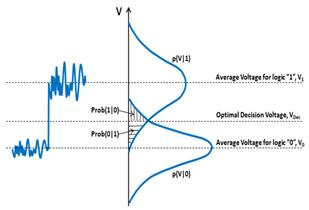Figure 6.2. Probability density functions of the voltage samples for the "1" and "0" logical values,"p(v|1)" and "p(v|0)", respectively.

Fig. 6.2 displays the fluctuations of the values of the voltage samples for the different logical levels. The decision circuit is responsible for comparing the value of each sample of the signal with a certain reference value, called decision voltage "VDec".

Still in fig. 6.2, the probability of the decision circuit to commit errors, can be shown. "Prob(1|0)" represents the probability that the decision circuit will decide for the logical value "1" when "0" was sent and, in turn, "Prob(0|1)" represents the probability that the decision circuit will decide for the logical value "0" when "1" was sent. Considering that the symbols are equiprobable and that the voltage of generated noise is described by a Gaussian distribution with zero mean value, the bit error probability is given by :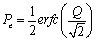(6.1)

where"erfc" is the complementary error function and "Q" is the quality factor. The "Q" factor is obtained from: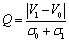(6.2)

where "V0" and "V1" are the average sample values for the "0" and "1" logical level, considering that the voltage samples were withdrawn at the optimum sampling instant of the signal and without inter-symbolic interference. On the other hand, "σ0" and "σ1", are the standard deviations of noise voltages for the different logical levels and which are obtained through the following expressions: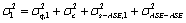(6.3)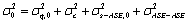(6.4)

where "σ2q,1" and "σ2q,0" represent the variance of the quantum noise voltage for the different logical levels, "σ2c" represents the variance of the noise circuit voltage, "σ2s-ASE,1" and , "σ2s-ASE,0" represent the voltage variance of the signal-ASE beat noise for the different logical levels and "σ2ASE-ASE" represents the voltage variance of the ASE-ASE beat noise.

The simulation of the optical receiver (Fig. 6.3) allows the incident optical signal to be configured on the receiver, the optical preamplifier and optical filter (if it is considered), the photodetector, the electrical amplifier and the equalizer.

The variable parameters of the incident optical signal and connector are made up by the average optical power that reaches the receiver, the path penalty, the wavelength, the Bit Rate, the extinction ratio and theoptical connector loss.

With relation to the optical preamplifier and the optical filter, the simulator allows scaling of its gain, noise figure and also the bandwidth of the optical filter.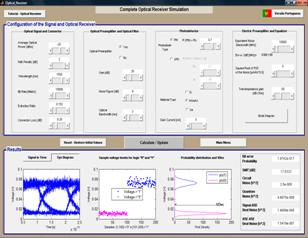Figure 6.3. optical receiver simulation interface.

With relation to the photodetector, the simulator allows for the choice of the type of photodiode, the scaling of its responsivity, its avalanche gain (in the case of the APD), the choice of the type of material it is made of, and also allows the quantification of the dark current that is present in the photodiode.

With relation to the parameters of the electrical amplifier and equalizer, the simulator allows the scaling of the bandwidth, the square root of the spectral density of noise power (a measure that is normally present in the catalogues of optical receivers) and the transimpedance gain. The simulator allows also, the display of the Bode diagram of the transfer function of the electrical preamplifier and equalizer, according to the introduced parameters.

In the results that are presented, the simulator presents graphically the resulting signal at the input of the decision circuit, as well as the corresponding eye diagram. Voltage samples will be presented, extracted at the optimal sampling instant for the different logical levels. It is possible, also, to display the probability density functions of the voltage samples, as well as the optimum decision voltage.The values resulting from the bit error probability, the value of the signal-to-noise ratio (bearing in account the penalty due to the extinction ratio) and the values of the different types of noise that are present in the obtained signal (circuit, quantum, signal-ASE beat and ASE-ASE beat noise), are also presented.

7. Final Conclusions, Perspectives of Future Work and Original Contributions

7.1. Final Conclusions

With relation to the developed software, it is to be stressed that it is fully original. There was a strong concern in creating a user-friendly graphical interface and, simultaneously, a functional interface.

Controllers were implemented which, given the physical limitations and theoretical considerations, prevents the simulator user from taking certain options, by presenting warnings and giving the possibility of automatic correction of these same options. The results are presented numerically and graphically for a better and speedier analysis of the same.

In order to increase the universe of potential users, the simulator was developed in two versions: Portuguese and English. Since the two versions are integrated into the simulator the change of version can be carried out in any of the windows displayed.

The present simulator has a very important pedagogical side to it, since, by means of the variation of the input parameters, the output signal can be displayed, thus providing a solid training about the operation of this type of device.

The simulator constitutes an alternative to the systems of real components in the following manners:

Economical – the conception and maintenance of real system laboratories is very expensive;

Quick – a simulation is performed in a few seconds, on real systems it could take days;

Portable – the simulations can be executed in any place with recourse to a single computer and its corresponding software;

Easy access – there is no need for access restrictions related with security and preservation of the integrity of real system laboratories.

Taking into consideration the proposed objectives of this present article, we can conclude that these were fully accomplished.

7.2. Perspectives of Future Work

This work may serve as a basis for future work in this so promising area.

An interesting task would be the development of a simulator for coherent optical systems, where the information is modulated into a carrier through its amplitude "ASK", frequency "FSK" or phase "PSK". In this case the optical receiver would become more complex, requiring a local optical oscillator (laser diode), were the output is duly "mixed" with the optical signal received, in order to obtain the information contained in the optical carrier.

Given the growing importance of optical communications in the world, the existence of this type of simulator is imperative, since it can contribute to a better understanding of the components of an optical communications receiver, improve the training capabilities and provide a friendly tool with easy upgrading for other types of components.

7.3. Original Contributions

The simulator developed for the IM-DD optical receiver contains several original contributions, as follows:

The graphical interface of the simulator was originally designed and implemented, following the strategies of Ben Schneidermann and using the available tools of the programming languageMatlab. This resulted in a functional and very user-friendly graphical interface.

For the simulation of the optical preamplifier a graphic was implemented which allows for the simultaneous display of the signal power and the noise power at the preamplifier output. This graphic gives the user a rapid perception of the quality of the signal.

In the simulation of the photodetector, the graphic of the response of the photodetector to the incident optical power was implemented. The graphic takes into account the noise and the impulsive response of the photodetector, according to the parameters inserted for the simulation.

The simulation of the electrical preamplifier and equalizer shows the Bode diagram of the different types of selected and scaled preamplifiers. An automatic scaling option of the equalizer was also implemented, in case the user intends to perform a perfect equalisation.

For the simulation of the complete optical receiver, in addition to the signal at the input of the decision circuit and the corresponding eye diagram, stand out the implementation of the graphic that allows for the display of the probability density functions of the samples of the different logical levels and, simultaneously, the optimum decision value. Stand out, also, the implementation of the calculation of the bit error probability of the receiver from the probability density functions of the samples, as well as the discriminated acquisition of the power of each of the different sources of noise.

Acknowledgments

The author would like to thank Prof.ª Maria João Marques Martins and Prof. José Luís Gonçalves Correia da Mata for their assistance.

References

1. GIOZZA, Willian F., CONFORTI, Evandro, WALDMAN, Hélio, "Fibras Ópticas – Tecnologia e Projeto de Sistemas", McGraw-Hill, São Paulo, Brasil, 1991
2. CARTAXO, A.V, Transmissão por fibra óptica, IST, Lisboa, Portugal, 2005
3. PIRES, J., "Sistemas de telecomunicações I", IST, Lisboa, Portugal, 1999
4. SMITH, David R., "Digital Transmission Systems", Third Edition, Kluwer Academic Publishers, USA, 2004
5. MARTINS, M. J., "Introdução às fibras ópticas", AEIST Press, 1997
6. MOLLENAUER, Linn F., GORDON, James P., "Optic Fibers- Fundamentals and Applications", Elsevier Academic Press, USA, 2006
7. GREEN, Paul E., "Fiber Optic Networks", Prentice Hall, New Jersey, USA, 1993
8. KEISER, Gerd, "Optical Fiber Communication", Third Edition, McGraw-Hill, Singapore, 2000
9. OLIVEIRA, Júlio César Rodrigues Fernandes, "Amplificadores Ópticos com Controle Automático de Ganho para Aplicação em Redes Ópticas Reconfiguráveis", Dissertação de Mestrado, Universidade Estadual de Campinas, 2007
10. PIRES, J., "Sistemas de Comunicação Óptica ", IST, Lisboa, Portugal, 1998
11. AGRAWAL, Goving P., "Fiber-Optic Communications Systems", Second Edition, Wiley-Interscience, USA, 1997
12. MARTINS, Maria João, PEREIRA, Marco Costa, "Estudo de DetectoresÓpticos com interesse para Aplicações Militares", Revista Proelium, IV Série nº4,pp.9-18,Academia Militar, Lisboa, 2006
13. AGRAWAL, "Goving P., "Lightwave Technology – Telecommunications Systems", Wiley-Interscience, New Jersey, USA, 2005
14. SALEH, B.E.A.,TEICH, M.C., "Fundamentals of Photonics", Wiley-Interscience, USA, 1991
15. CVIJETIC, Milorad, "Optical Transmission Systems Engineering", Artech House, Norwood, USA, 2004
16. MARTINS, Maria João, GARRIDO, P, ALMEIDA,V., "Desenvolvimento de um Simulador de Fibra Óptica", Revista Proelium, VI Série nº8, Academia Militar, Lisboa, 2007
17. KAZOVSKY, Leonid, BENEDETTO, Sergio, WILLNER, Alan, "Optical Fiber Communication Systems", Artech House, Norwood, USA, 1996
18. SHNEIDERMAN, Ben, "Designing the User Interface: Strategies for Effective Human-Computer Interaction", Addison-Wesley , Massachusetts,1992
19. (http://www.mathworks.com/)(September 15, 2009).

 Contents 1. 2. 2.1. 2.2. 3. 3.1. 3.2. 3.3. 3.4. 4. 4.1. 4.2. 4.3. 4.4. 4.5. 5. 5.1. 5.2. 5.3. 5.4. 6. 6.1. 6.2. 7. 7.1. 7.2. 7.3.
Article ToolsAbstractPDF(1962K)